# OOF2: The Manual

## Name

4th order Runge-Kutta (RK4) — Fourth order Runge-Kutta time stepping.

## Synopsis

RK4()

## Description

RK4 is a TimeStepper that implements the classic fourth order Runge-Kutta method for solving ordinary differential equations. The error on each step is of order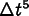.

Given a vector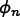of unknowns (i.e. Field values in OOF2) at time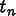, and the first order differential equation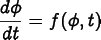(6.157)

the fourth order Runge-Kutta estimate for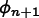is given by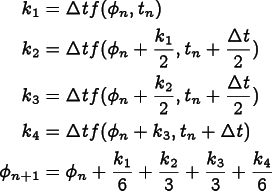(6.158)

where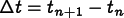.

RK4 can be applied to second order equations by using equation (6.141).

Because the method is explicit (doesn't appear as an argument to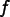), equation (6.158) doesn't require a nonlinear solver even ifis nonlinear.